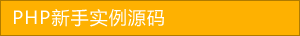﻿ 又一个PHP实现的冒泡排序算法分享 - php应用 - PHP粉丝网

# 又一个PHP实现的冒泡排序算法分享

##### 发布:smiling　来源: PHP粉丝网 　添加日期：2021-04-07 16:25:42　浏览: 评论：0

1. <?php
2. /**
3.  * PHP中的冒泡排序法使用
4.  */
5.
6. // 预先声明一个数组
7. \$arr = array (12,45,28,30,88,67);
8. echo "原数组"
9. print_r(\$arr);
10. echo "<br/>"
11. //冒泡排序
12. function maopao(\$arr){
13.   // 进行第一层遍历
14.   for(\$i=0,\$k=count(\$arr);\$i<\$k;\$i++) {
15.     // 进行第二层遍历 将数组中每一个元素都与外层元素比较
16.     // 这里的i+1意思是外层遍历当前元素往后的
17.     for (\$j=\$i+1;\$j<\$k;\$j++) {
18.       // 内外层两个数比较
19.         if(\$arr[\$i]<\$arr[\$j]){
20.         // 先把其中一个数组赋值给临时变量
21.           \$temp = \$arr[\$j];
22.         // 交换位置
23.         \$arr[\$j] = \$arr[\$i];
24.         // 再从临时变量中赋值回来
25.         \$arr[\$i] = \$temp
26.       }
27.     }
28.   }
29.   // 返回排序后的数组
30.   return \$arr
31.
32. // 直接打印排序后的数组
33. echo '排序后'
34. print_r(maopao(\$arr));
35.
36. ?>

Array (  => 12  => 45  => 28  => 30  => 88  => 67 )

Array (  => 88  => 67  => 45  => 30  => 28  => 12 )

## 相关文章